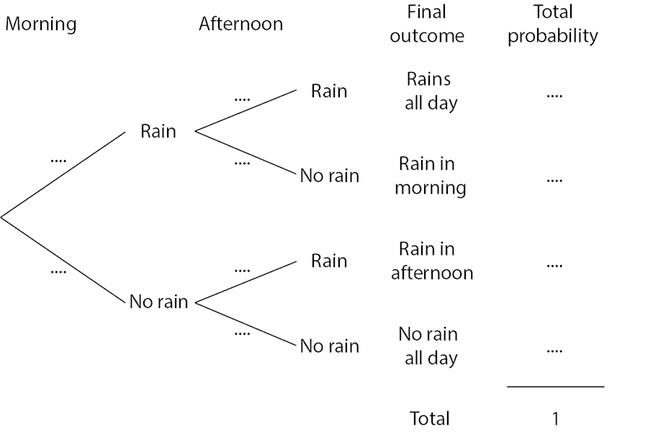## Want to keep learning?

This content is taken from the Cambridge University Press's online course, Teaching Probability. Join the course to learn more.
3.13

## Cambridge University PressProbability tree diagram for question 3

# Two non-independent events

When events are not independent, we need to take into account ‘what has already happened’ when deciding what value of probability to use for subsequent events.

More formally, we can no longer use the simple multiplication rule for independent events $$X$$ and $$Y$$:

$p(X\cap Y)=p(X).p(Y)$

Instead, when $$X$$ and $$Y$$ are not independent, we need to multiply the probability of $$X$$ by the probability of $$Y$$ given that $$X$$ has occurred:

$$p(X\cap Y)=p(X).p(Y$$|$$X)$$

Probability tree diagrams are often especially useful for tackling this kind of problem.

## Sample examination-style questions

### Question 3

The probability that it rains in the morning is 0.3. If it rains in the morning, the probability that it carries on raining in the afternoon is 0.5. If it does not rain in the morning, the probability that it carries on being fine in the afternoon is 0.9.

• Copy and complete the probability tree diagram (above) using this information.

• What is the probability that the weather is the same in the afternoon as in the morning?

• If it rained during the day, what is the probability that it rained in the morning?

### Question 4

Adam has 3 white single socks and 4 black single socks that he keeps loose in a drawer.

• If he takes out two socks at random in the morning, what is the probability he picks a pair of socks of the same colour?

### Question 5

Louis has 10 sweets in a bag, a mixture of toffees and chocolates. Sophia takes two sweets at random and eats them. The probability that Sophia eats two chocolates is $$\frac{1}{3}$$.

• How many chocolates were in the bag?

There are some ‘old favourites’ here. Notice that question 5 is reminiscent of another problem, which we looked at in week 2! Notice also that the question numbers run on from the previous step; we will provide all of our solutions to these questions after the next step.